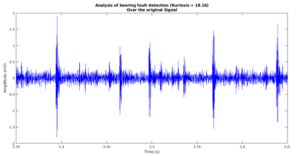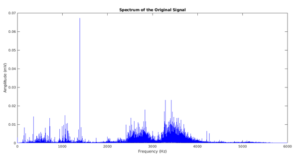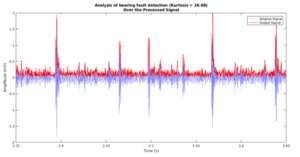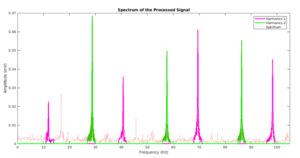1693 Order Completed
92 % Response Time
87 Reviews
Since 2014

# Magnetic Analysis Engineer

## Samuel P

Master’s Degree, Electrical Engineering, University of Waterloo, Canada

Profession

Experienced electrical engineering assignment help expert

Skills

I have a Master’s degree in electrical engineering and close to 7 years of experience in electromagnetics, radar sensors, magnetic analysis, 3D electromagnetic modeling, and digital image processing. I also have a vast knowledge of fault analysis systems, password-based circuit breakers, temperature controllers, and alternating current machines. Since 2014, I have been offering academic assistance with these topics to help students who are struggling with projects in these areas.

Get Free Quote
0 Files Selected

Kurtosis Coefficient and Frequency Spectrum   We have applied the function developed on the data set 172.mat downloaded from here. This data set corresponds to a signal of bearing faults at a speed rate of 1730 RPM, obtained at a sampling rate of 12000 samples/second (Fs), and a load defined as load 3. We have used 2 Hz and 4 kHz as cutoff frequencies for the bandpass filter. Next, we show the original signal with its kurtosis coefficient (Fig 2.a) and the frequency spectrum (Fig 2.b).Figure 2. (a) Time series original signal. (b) Spectrum

We have limited the time axis to better distinguish the distortions that induce faults. We see that in this domain you can observe such faults and therefore your kurtosis coefficient is relatively large. However, in the domain of frequency we observe what was expected, it is not easy to appreciate the Fo components and their harmonics. We observe a strong spectrum contaminated by many frequency components. In other words, we can not find the components of interest, such components are lost. In Figure 3 we can see the enveloping signals with their kurtosis coefficient (Fig 3.a, processed signal) and their spectrum in the frequency domain.Figure 2. (a) Time series original signal. (b) Spectrum

From figure 3.a we can see how the procedure followed, in effect, is able to determine the envelope of the signal, resulting in a signal processing envelope surrounding the original signal. We can see how the kurtosis coefficient increases considerably which makes the signal more feasible for the detection of faults. This signal in theory would help us to determine the Fo components and their harmonics because it captures the information of the distortions while attenuating the other distortions that are not of interest. This signal is already prepared to make a spectral analysis   Precisely, Figure 3.b shows the spectrum of the envelope signal. We observe in this one how there is a strong concentration of components at low frequencies. It is in this region where the Fo components and their harmonics lie. It is worth doing a zoom in low frequencies to detail such a region. This is exactly what figure 4 shows.Figure 4. Zoom at low frequencies

In this figure also zoom in low frequencies, we have taken the liberty to highlight what seems to be the components of two bearing failures. One of them we have colored in green and the other in magenta. We observe how both are equally spaced and assume that it is due to the fundamental frequency and its harmonics.
CONCLUSIONS
A function written in MATLAB was developed to perform analyzes on bearing failure signals using a basic processing technique known as envelope analysis. This function was applied to a set of real data and its optimal functioning was verified by detecting the frequency components related to the faults (fundamental component and its harmonics). This function is generalizable. In fact, it can be applied to any set of bearing signal data to obtain output arguments with which a complete analysis can be made. You just have to be careful to properly define the input parameters such as the cutoff frequencies of the bandpass filter and the signal sampling frequency.